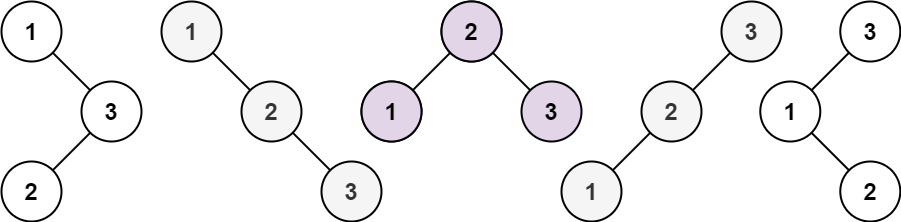# Unique Binary Search Trees LeetCode Solution

Unique Binary Search Trees LeetCode Solution says that – Given an integer `n`, return the number of structurally unique BST’s (binary search trees) which has exactly `n` nodes of unique values from `1` to `n`.

Example 1:Input:

``` n = 3
```

Output:

``` 5
```

Example 2:

Input:

``` n = 1
```

Output:

``` 1
```

Constraints:

• `1 <= n <= 19`

## Algorithm Of Unique Binary Search Trees LeetCode Solution –

### IDEA –

In this problem, we are asked to get the number of trees and not necessarily to return all trees, only the number. Here we can use the idea of dynamic programming, let `dp[n]` be the number of unique Binary Search Trees with `n` nodes. How can we evaluate them: we need to choose the number of nodes in the left subtree and the number of nodes in the right subtree, for example `n=5`, then we have options:

1. The left subtree has 0 nodes, root = 1, and the right subtree has 4 nodes, a number of options `f*f`
2. The left subtree has 1 node, root = 2, and the right subtree has 3 nodes, a number of options `f*f`
3. The left subtree has 2 nodes, root = 3, and the right subtree has 2 nodes, number of options `f*f`
4. The left subtree has 3 nodes, root = 4, and the right subtree has 1 node, number of options `f*f`
5. The left subtree has 4 nodes, root = 5, and the right subtree has 0 nodes, number of options `f*f`

So, in total `f = f*f + f*f + f*f + f*f + f*f`, and in general:
`f[n] = f*f[n-1] + f*f[n-2] + ... + f[n-2]*f + f[n-1]*f`.

• In order to find Unique Binary Search Trees. we will use the concept of the Catalan numbers in this question we will use formulas like – C2 = C0*C1 + C1*C0.

C3 = C0*C2 + C1*C1 + C2*C0.

C4 = C0*C3 + C1*C2 + C2*C1 + C3*C0.

• At last, we will return the C-th number. we will use the Catalan number in this question to find the unique number of BST. There are lots of applications for Catalan numbers.

### APPROACH –

• At first, we will create one array of length n+1 and initially, the value of the first index will be 1.
• After that, we will run a loop from 1 to n+1 and within the loop, we will run a nested loop from 0 to i.
• Within the loop, we will update the i-th position of the array by adding the multiplication of the respective index and at last, we will return the value of the nth index of the array.
• Hence we will find the Unique Binary Search Tree.

### IMAGE OF THE SOLUTION –```class Solution {
public int numTrees(int n) {
int[] res = new int[n+1];
res= 1;
for(int i=1; i <=n; i++)
for(int j = 0; j<i; j++)
res[i] += res[j]*res[i-j-1];
return res[n];
}
}```
```class Solution:
def numTrees(self, n: int) -> int:
res = *(n+1)
res = 1
for i in range(1,n+1):
for j in range(i):
res[i] += res[j]*res[i-j-1]
return res[n]```

TIME COMPLEXITY – O(N^2), As we have used a nested loop here.

SPACE COMPLEXITY – O(N), As we have created one array on length ‘n’.

Translate »